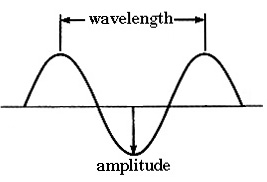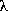Spectroscopy - Properties of Light

## Properties of Light

### Light

Light is actually a form of electromagnetic radiation. Electromagnetic radiation can be thought of as a wave having both magnetic and electrical components. A wave can be described by its various characteristics.crests and troughs the high and low points of a wave, respectively speed how fast a wave moves in a given direction the speed of light in a vacuum (c) is 3 x 108 m/s wavelength () the distance between two consecutive crests or troughs review the appropriate metric prefixes frequency (v) the number of waves that pass a given point in a given time period the unit for frequency is hertz (sec-1) for light waves c = v *amplitude the "height" of a wave energy (E) the energy of one photon of light E = h * v (where h is Planck's constant = 6.626 x 10-34 J.s)